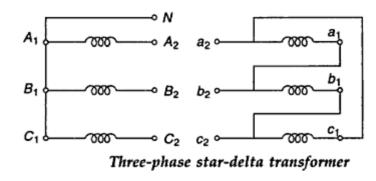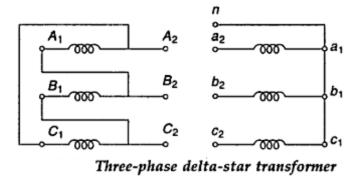### Three Phase Transformer Connection

The following 4 ways of connecting the 3-phase transformers will be discussed here.
• Delta - Delta (∆ - ∆)
• Star - Star ( Y - Y )
• Delta - Star (∆- Y)
• Star - Delta (Y - ∆)
In 3-phase systems, the above connections may be made by banks of suitably connected single phase transformers in which the magnetic circuit of three phases is inter linked.
1. Star - Star connection
- In Star - Star connection both the primary and secondary windings are connected in Star.
- The neutral point is denoted by N for high voltage winding and n for low voltage winding and the connection is shown in Fig.1.
- The phase current is equal to the line current but the line voltage is (√3) times the phase voltage in both the primary and secondary windings.
- Under balanced load conditions, whether the neutrals are earthed or unearthed.
IA + IB + IC = 0 and  Ia + Ib + Ic = 0
Where IA , IB and IC refer to primary side Ia + Ib and Ic to secondary.
- The Star - Star connection is not used in a three phase three wire system due to undesirable effects of a third harmonic current.Fig.  1

2. Delta - Delta connection
- In delta connection both the primary and secondary windings are connected in Delta as shown in Fig. 2.
- Here the line voltage is equal to phase voltage on each side and the phase current is line current divided by (√3).
- As compared to a star - star connection for the same terminal voltage and current, a delta - delta connection has more number of turns in each phase winding but less cross-section area of conductors. Hence a delta connection is more economical for large transformers of relatively lower voltage rating.
- The 3rd harmonic exciting current will circulate within the closed ∆ and shall not appear on the line.
- The ∆ connection also acts as a block to the flow of Zero-sequence currents.
- The ∆ closed serves to damp out third harmonic voltages.
- It is possible to operate the transformer on 58% of its normal rating in V-connection if one of the phases develop fault.
- The absence of star-point may be disadvantageous. If one line gets earthed due to fault, maximum voltage between windings and the core will be full line voltage.Fig.  2

3. Star - Delta connection
- Fig. 3 shows the connection for a three phase Star - delta transformer.
- On the primary side the line voltage is ( √3) times the phase voltage while the line and phase voltages are equal on the secondary side.
- Generally, the high voltage winding is star connected for reducing cost of insulation.
- This connection is generally used for step down transformers at receiving end substations.Fig  3

3. Delta - Star connection
- The connection of a three phase delta - star transformer is shown in Fig. 4.
- On the primary side the line and phase voltages are equal, but on the secondary side the line voltage is ( √3) times the phase voltage
- It has the advantage of Y-point for mixed loading and winding to carry third harmonic currents, which stabilize the star point potential.
- These transformers are used at sending and receiving end substations. At power stations the generator feeds the delta winding and the star winding is connected to h.v. transmission lines. In distribution transformers, feeders are connected to delta winding and the star winding supplies three-phase four-wire distributors.Fig.  4